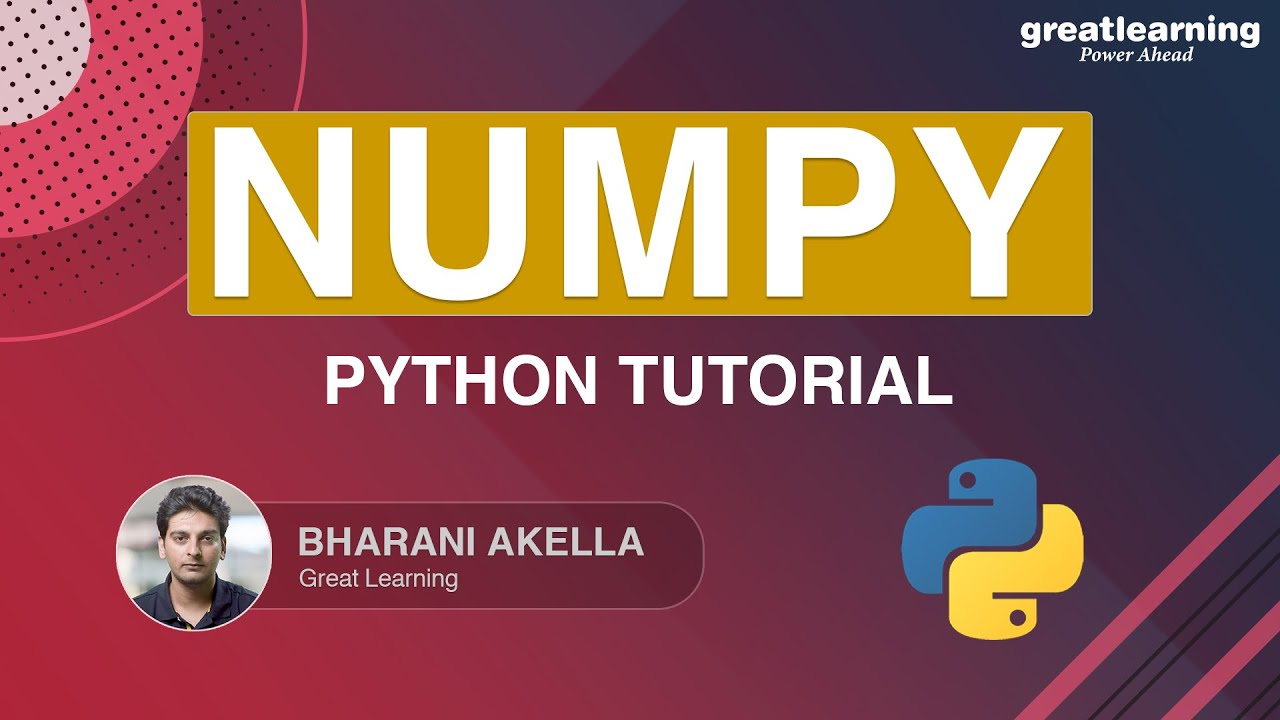# Numpy Python Tutorial | Python TrainingNumPy (Numerical Python) is a Python library that is used for working with arrays. NumPy provides an array object which is known as “ndarray” and the fun fact is, it’s 50x faster than lists. NumPy is a Python library and is written partially in Python, but most of the parts that require fast computation are written in C or C++. To know more about this interesting library with amazing examples, watch this tutorial till the end.

NumPy is a python library that is used for working with arrays. NumPy was developed by Travis Oliphant in 2005. It is an open source project and you can use it freely. NumPy stands for Numerical Python. It is helpful in solving problems related to linear algebra, fourier transform, and matrices.

One of the most common Python data structures is lists that serve the purpose of arrays, but they are slow to process. NumPy provides an array object which is known as “ndarray” and the fun fact is, it’s 50x faster than lists.

NumPy arrays are stored at one continuous place in memory unlike lists, so processes can access and manipulate them very efficiently. NumPy is a Python library and is written partially in Python, but most of the parts that require fast computation are written in C or C++. To know more about this interesting library with amazing examples, watch this tutorial till the end.

Following pointers will be covered in this video:

• 00:00:00 - Introduction
• 00:00:41 Python Numpy
• 00:01:07 Creating NumPy Array
• 00:11:44 NumPy-Shape
• 00:14:02 Joining NumPy Arrays
• 00:17:34 NumPy Intersection & Difference
• 00:21:08 Numpy Array Mathematics
• 00:28:35 NumPy Matrix
• 00:31:47 NumPy Matrix Transpose
• 00:32:42 NumPy Matrix Multiplication
• 00:35:05 NumPy Save & Load

## Hire Machine Learning Developers in India

We supply you with world class machine learning experts / ML Developers with years of domain experience who can add more value to your business.

## Hire Python Developers

Are you looking for experienced, reliable, and qualified Python developers? If yes, you have reached the right place. At **[HourlyDeveloper.io](https://hourlydeveloper.io/ "HourlyDeveloper.io")**, our full-stack Python development services...

## Hire Python Developers India

Looking to build robust, scalable, and dynamic responsive websites and applications in Python? At **[HourlyDeveloper.io](https://hourlydeveloper.io/ "HourlyDeveloper.io")**, we constantly endeavor to give you exactly what you need. If you need to...

## Python Tutorial - Learn Python for Machine Learning and Web Development

Learn Python for Machine Learning and Web Development. Can Python be used for machine learning? Python is widely considered as the preferred language for teaching and learning ML (Machine Learning). Can I use Python for web development? Python can be used to build server-side web applications. Why Python is suitable for machine learning? How Python is used in AI? What language is best for machine learning?

## How To Plot A Decision Boundary For Machine Learning Algorithms in Python

How To Plot A Decision Boundary For Machine Learning Algorithms in Python, you will discover how to plot a decision surface for a classification machine learning algorithm.